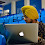# CSE205: Data Structures and Algorithms CA-1 ( Class Test ) Lovely Professional University

Course Outcomes:
• develop skills to design and analyze linear and non linear data structures
• assess how the choice of data structures and algorithm design methods impacts the performance of programs
• identify and apply the suitable data structure for the given real world problem

CSE205:DATA STRUCTURES AND ALGORITHMS L:3 T:1 P:0 Course Outcomes: • develop skills to design and analyze linear and non linear data structures • assess how the choice of data structures and algorithm design methods impacts the performance of programs • identify and apply the suitable data structure for the given real world problem Unit I Introduction : Basic Data Structures, Basic Concepts and Notations, Complexity analysis: time space and trade off, Omega Notation, Theta Notation, Big O notation Arrays : Linear arrays: memory representation, Traversal, Insertion, Deletion, Searching, Merging and their complexity analysis. Sorting and Searching : Bubble sort, Insertion sort, Selection sort Unit II Linked Lists : Introduction, Memory representation, Allocation, Traversal, Insertion, Deletion, Header linked lists: Grounded and Circular, Two-way lists: operations on two way linked lists Unit III Stacks : Introduction: List and Array representations, Operations on stack (traversal, push and pop), Arithmetic expressions: polish notation, evaluation and transformation of expressions., Evaluation and transformation of expressions, Towers of Hanoi, Merge sort Queues and Recursion : Array and list representation, operations (traversal, insertion and deletion), Priority Queues, Deques, Function Call, Recursion implementation and Complexity issues. Unit IV Trees : Binary trees: introduction (complete and extended binary trees), memory representation (linked, sequential), Pre-order traversal using Stack, In-order traversal using Stack, Post-order traversal using Stack, Binary Search Treesearching, Binary Search Tree- Insertion, Binary Search Tree- deletion Unit V AVL trees and Heaps : AVL trees Introduction, AVL trees Insertion, AVL trees Deletion, Heaps: Insertion, Heaps: Deletion, HeapSort, Huffman algorithm Unit VI Graphs : Warshall's algorithm, Shortest path algorithm Floyd Warshall Algorithm (modified warshall algorithm), Graph Traversal: BFS, DFS Hashing : Hashing Introduction, Hash Functions, Hash Table, Closed hashing (open addressing), Linear Probing, Quadratic Probing, Double Hashing, Open hashing (separate chaining) Text Books: 1. DATA STRUCTURES by SEYMOUR LIPSCHUTZ, MCGRAW HILL EDUCATION References: 1. DATA STRUCTURES AND ALGORITHMS by ALFRED V. AHO, JEFFREY D. ULLMAN AND JOHN E. HOPCROFT, PEARSON Credits:4 Through this course students should be able to Page:1/1 TermID : 18191

1.2.